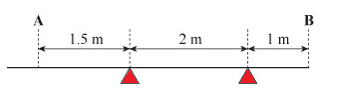# Beam calculation with 3 unknowns

## Homework Statement

A 5m metal beam is supported on 2 supports. The beam is 30kg and the man is 65kg. The man stands at A, to prevent the beam tipping extra weights have been placed at B. Find reaction forces and the weights that have been placed at B. Take acceleration due to gravity to be 9.8ms## Homework Equations

Force * Distance
Sum of Clockwise = Sum of Anti-Clockwise
Sum of Downward Forces = Sum of Upward Forces

## The Attempt at a Solution

Decorator - 637N
Beam - 294N
F1 being the first support reaction force
F2 being the second reaction force
W being the extra weights added

F1 + F2 = 931 + W (Sum of up and down)

3F1 + F2 = 3601.5 (sum of clockwise/anticlockwise)

I have attempted so many different ways to figure this out, but I have no idea how to get around having 3 unknowns at a time. When I take a moment around one of the supports, I have the reaction force the other plus the weight being placed at B. I'm not sure if I'm missing something obvious or not.
Thanks in advance for literally any help

#### Attachments

TSny
Homework Helper
Gold Member
Welcome to PF!

Maybe you are supposed to assume that the weight at B is the minimum weight that will prevent the beam from tipping.

I don't quite agree with your number 3601.5 in the torque equation. EDIT: Nevermind, I see that A is not at the end of the beam. The number 3601.5 looks good.

Last edited:
Ray Vickson
Homework Helper
Dearly Missed

## Homework Statement

A 5m metal beam is supported on 2 supports. The beam is 30kg and the man is 65kg. The man stands at A, to prevent the beam tipping extra weights have been placed at B. Find reaction forces and the weights that have been placed at B. Take acceleration due to gravity to be 9.8ms
View attachment 226011

## Homework Equations

Force * Distance
Sum of Clockwise = Sum of Anti-Clockwise
Sum of Downward Forces = Sum of Upward Forces

## The Attempt at a Solution

Decorator - 637N
Beam - 294N
F1 being the first support reaction force
F2 being the second reaction force
W being the extra weights added

F1 + F2 = 931 + W (Sum of up and down)

3F1 + F2 = 3601.5 (sum of clockwise/anticlockwise)

I have attempted so many different ways to figure this out, but I have no idea how to get around having 3 unknowns at a time. When I take a moment around one of the supports, I have the reaction force the other plus the weight being placed at B. I'm not sure if I'm missing something obvious or not.
Thanks in advance for literally any help

You say the beam is 5m in length, but the segments shown add up to only 4.5m. Which figure is erroneous?

TSny
Homework Helper
Gold Member
You say the beam is 5m in length, but the segments shown add up to only 4.5m. Which figure is erroneous?
I overlooked that A is not at the end of the beam. So, the beam can be 5 m long.

You say the beam is 5m in length, but the segments shown add up to only 4.5m. Which figure is erroneous?
The beam is 5m in length, the man is standing 0.5m away from the end of the beam.

haruspex